Next: Ionospheric Ray Tracing Up: Wave Propagation in Inhomogeneous Previous: Ionospheric Pulse Propagation

# Measurement of Ionospheric Electron Density Profile

The equivalent height of the ionosphere can be measured in a fairly straightforward manner, by timing how long it takes a radio pulse fired vertically upwards to return to ground level again. We can, therefore, determine the function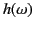experimentally by performing this procedure many times with pulses of different central frequencies. But, is it possible to use this information to determine the number density of free electrons in the ionosphere as a function of height? In mathematical terms, the problem is as follows. Does a knowledge of the function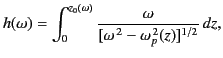(1131)

where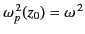, necessarily imply a knowledge of the function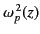? Recall that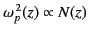.

Let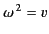and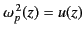. Equation (1133) then becomes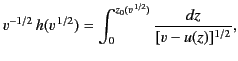(1132)

where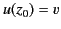, andfor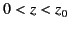. Let us multiply both sides of the previous equation by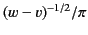and integrate from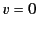to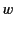. We obtain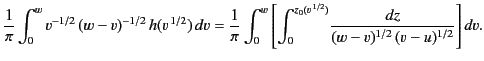(1133)

Consider the double integral on the right-hand side of the previous equation. The region of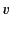-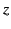space over which this integral is performed is sketched in Figure 782. It can be seen that, as long as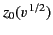is a monotonically increasing function of, we can swap the order of integration to give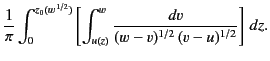(1134)

Here, we have used the fact that the curve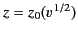is identical with the curve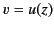. Note that ifis not a monotonically increasing function ofthen we can still swap the order of integration, but the limits of integration are, in general, far more complicated than those indicated previously. The integral overin the previous expression can be evaluated using standard methods (by making the substitution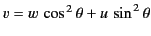): the result is simply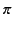. Thus, expression (1136) reduces to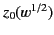. It follows from Equation (1135) that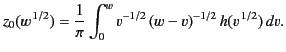(1135)

Making the substitutions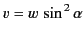and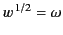, we obtain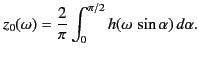(1136)

By definition,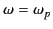at the reflection level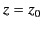. Hence, the previous equation reduces to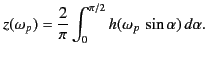(1137)

Thus, we can obtainas a function of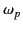(and, hence,as a function of) by simply taking the appropriate integral of the experimentally determined function. Because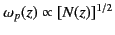, this means that we can determine the electron number density profile in the ionosphere provided that we know the variation of the equivalent height with pulse frequency. The constraint that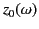must be a monotonically increasing function of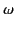translates to the constraint that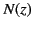must be a monotonically increasing function of. Note that we can still determinefromfor the case where the former function is non-monotonic, it is just a far more complicated procedure than that outlined previously. Incidentally, the mathematical technique by which we have inverted Equation (1133), which specifiesas some integral over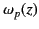, to giveas some integral over, is known as Abel inversion.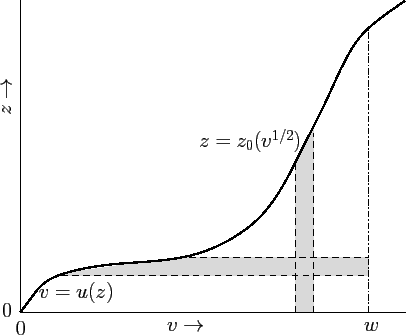Next: Ionospheric Ray Tracing Up: Wave Propagation in Inhomogeneous Previous: Ionospheric Pulse Propagation
Richard Fitzpatrick 2014-06-27